# scatterhist

Scatter plot with marginal histograms

## Syntax

``scatterhist(x,y)``
``scatterhist(x,y,Name,Value)``
``h = scatterhist(___)``

## Description

example

````scatterhist(x,y)` creates a 2-D scatter plot of the data in vectors `x` and `y`, and displays the marginal distributions of `x` and `y` as univariate histograms on the horizontal and vertical axes of the scatter plot, respectively.```

example

````scatterhist(x,y,Name,Value)` creates the plot using additional options specified by one or more name-value pair arguments. For example, you can specify a grouping variable or change the display options.```

example

````h = scatterhist(___)` returns a vector of three axis handles for the scatter plot, the histogram along the horizontal axis, and the histogram along the vertical axis, respectively, using any of the input arguments in the previous syntaxes.```

## Examples

collapse all

Load the sample data. Create data vector `x` from the first column of the data matrix, which contains sepal length measurements from iris flowers. Create data vector `y` from the second column of the data matrix, which contains sepal width measurements from the same flowers.

```load fisheriris x = meas(:,1); y = meas(:,2);```

Create a scatter plot and two marginal histograms to visualize the relationship between sepal length and sepal width.

`scatterhist(x,y)`Display a data tip for a bin in a histogram. A data tip appears when you hover over a bin in a histogram.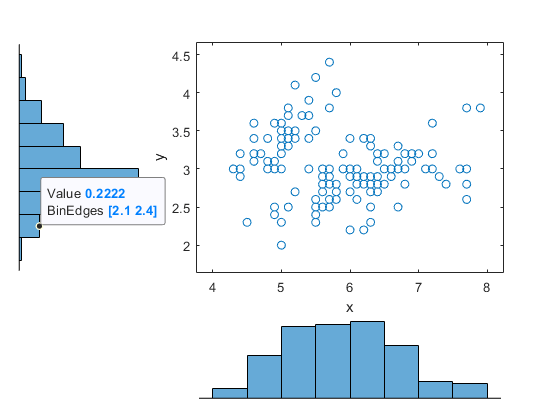The data tip displays the probability density function estimate of the selected bin and the lower and upper values for the bin edges.

Load the sample data. Create data vector `x` from the first column of the data matrix, which contains sepal length measurements from three species of iris flowers. Create data vector `y` from the second column of the data matrix, which contains sepal width measurements from the same flowers.

```load fisheriris.mat; x = meas(:,1); y = meas(:,2);```

Create a scatter plot and six kernel density plots to visualize the relationship between sepal length and sepal width, grouped by species.

`scatterhist(x,y,'Group',species,'Kernel','on')`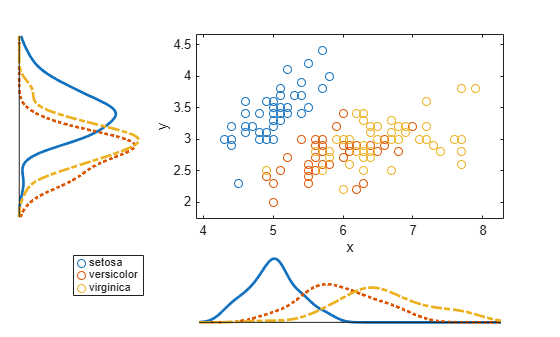The plot shows that the relationship between sepal length and width varies depending on the flower species.

Load the sample data. Create data vector `x` from the first column of the data matrix, which contains sepal length measurements from three different species of iris flowers. Create data vector `y` from the second column of the data matrix, which contains sepal width measurements from the same flowers.

```load fisheriris.mat; x = meas(:,1); y = meas(:,2);```

Create a scatter plot and six kernel density plots to visualize the relationship between sepal length and sepal width as measured on three species of iris flowers, grouped by species. Customize the appearance of the plots.

```scatterhist(x,y,'Group',species,'Kernel','on','Location','SouthEast',... 'Direction','out','Color','kbr','LineStyle',{'-','-.',':'},... 'LineWidth',[2,2,2],'Marker','+od','MarkerSize',[4,5,6]);```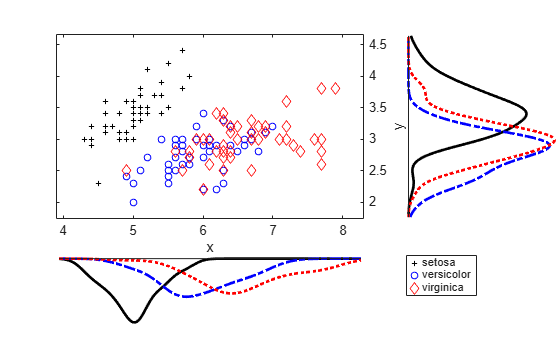Load the sample data. Create data vector `x` from the first column of the data matrix, which contains sepal length measurements from three species of iris flowers. Create data vector `y` from the second column of the data matrix, which contains sepal width measurements from the same flowers.

```load fisheriris.mat; x = meas(:,1); y = meas(:,2); ```

Use axis handles to replace the marginal histograms with box plots.

```h = scatterhist(x,y,'Group',species); hold on; clr = get(h(1),'colororder'); boxplot(h(2),x,species,'orientation','horizontal',... 'label',{'','',''},'color',clr); boxplot(h(3),y,species,'orientation','horizontal',... 'label', {'','',''},'color',clr); set(h(2:3),'XTickLabel',''); view(h(3),[270,90]); % Rotate the Y plot axis(h(1),'auto'); % Sync axes hold off; ```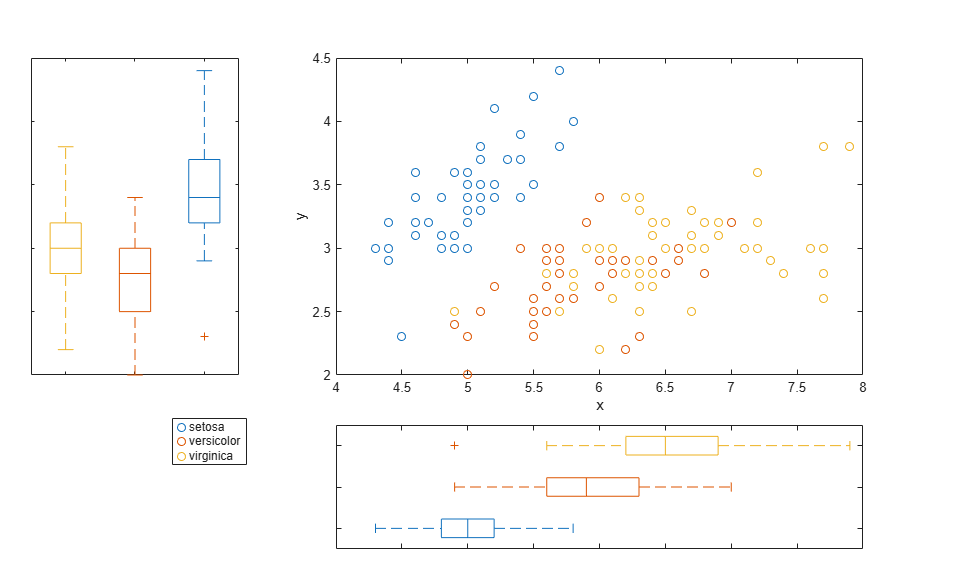Load the sample data. Create data vector `x` from the first column of the data matrix, which contains sepal length measurements from iris flowers. Create data vector `y` from the second column of the data matrix, which contains sepal width measurements from the same flowers.

```load fisheriris x = meas(:,1); y = meas(:,2);```

Create a new figure and define two `uipanel` objects to divide the figure into two parts. In the upper half of the figure, plot the sample data using `scatterhist`. Include marginal kernel density plots grouped by species. In the lower half of the figure, plot a histogram of the sepal length measurements contained in `x`.

```figure hp1 = uipanel('position',[0 .5 1 .5]); hp2 = uipanel('position',[0 0 1 .5]); scatterhist(x,y,'Group',species,'Kernel','on','Parent',hp1); axes('Parent',hp2); hist(x);```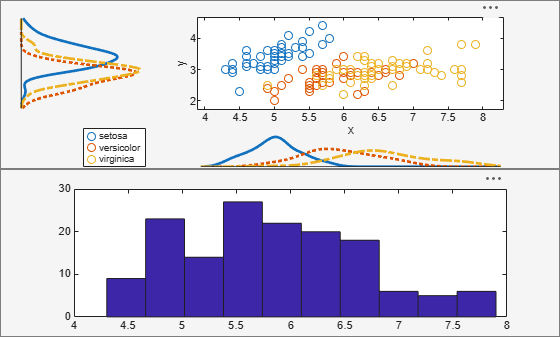## Input Arguments

collapse all

Sample data, specified as a vector. The data vectors `x` and `y` must be the same length.

If `x` or `y` contain `NaN` values, then `scatterhist`:

• Removes rows with `NaN` values in either `x` or `y` from both data vectors when generating the scatter plot

• Removes rows with `NaN` values only from the corresponding `x` or `y` data vector when generating the marginal histograms

Data Types: `single` | `double`

Sample data, specified as a vector. The data vectors `x` and `y` must be the same length.

If `x` or `y` contain `NaN` values, then `scatterhist`:

• Removes rows with `NaN` values in either `x` or `y` from both data vectors when generating the scatter plot

• Removes rows with `NaN` values only from the corresponding `x` or `y` data vector when generating the marginal histograms

Data Types: `single` | `double`

### Name-Value Arguments

Specify optional pairs of arguments as `Name1=Value1,...,NameN=ValueN`, where `Name` is the argument name and `Value` is the corresponding value. Name-value arguments must appear after other arguments, but the order of the pairs does not matter.

Before R2021a, use commas to separate each name and value, and enclose `Name` in quotes.

Example: `'Location','SouthEast','Direction','out'` specifies a plot with histograms located below and to the right of the scatter plot, with the bars directed away from the scatter plot.

Number of bins for histograms, specified as the comma-separated pair consisting of `'NBins'` and a positive integer value greater than or equal to 2, or vector of two such values. If the number of bins is specified as a positive integer value, that value is the number of bins for both the `x` and `y` histograms. If the number of bins is specified by a vector, the first value is the number of bins for the `x` data, and the second value is the number of bins for the `y` data. By default, the number of bins is computed based on the sample standard deviation using Scott’s rule.

Example: `'NBins',[5,7]`

Data Types: `single` | `double`

Location of the marginal histograms in the figure, specified as the comma-separated pair consisting of `'Location'` and one of the following.

 `'SouthWest'` Plot the histograms below and to the left of the scatter plot. `'SouthEast'` Plot the histograms below and to the right of the scatter plot. `'NorthEast'` Plot the histograms above and to the right of the scatter plot. `'NorthWest'` Plot the histograms above and to the left of the scatter plot.

Example: `'Location','SouthEast'`

Direction of the marginal histograms, specified as the comma-separated pair consisting of `'Direction'` and one of the following.

 `'in'` Plot the histograms with the bars directed toward the scatter plot. `'out'` Plot the histograms with the bars directed away from the scatter plot.

Example: `'Direction','out'`

Grouping variable, specified as the comma-separated pair consisting of `'Group'` and a categorical array, logical or numeric vector, character array, string array, or cell array of character vectors. Each unique value in a grouping variable defines a group.

For example, if `Gender` is a cell array of character vectors with values `'Male'` and `'Female'`, you can use `Gender` as a grouping variable to plot your data by gender.

The number of rows in the grouping variable must be equal to the length of `x`.

Example: `'Group',Gender`

Data Types: `categorical` | `single` | `double` | `logical` | `char` | `string` | `cell`

Grouped plot indicator, specified as the comma-separated pair consisting of `'PlotGroup'` and one of the following.

 `'on'` Display grouped histograms or grouped kernel density plots. This is the default if a `Group` parameter is specified. `'off'` Display histograms or kernel density plots of the whole data set. This is the default if a `Group` parameter is not specified.

Example: `'PlotGroup','off'`

Histogram display style, specified as the comma-separated pair consisting of `'PlotGroup'` and one of the following.

 `'stairs'` Display a stairstep plot that shows the outline of the histogram without filling the bars. This is the default if you specify a grouping variable that contains more than one group. `'bar'` Display a histogram bar plot. This is the default if you specify a grouping variable that contains only one group or if `PlotGroup` is specified as `'off'`.

Example: `'Style','bar'`

Kernel density plot indicator, specified as the comma-separated pair consisting of `'Kernel'` and one of the following.

 `'off'` Display the marginal distributions as histograms. `'on'` Display the marginal distributions as kernel density plots. `'overlay'` Display the marginal distributions as kernel density plots overlaid onto histograms, similar to `histfit`.

Example: `'Kernel','overlay'`

Bandwidth of kernel smoothing window, specified as the comma-separated pair consisting of `'Bandwidth'` and a matrix of size 2-by-K, where K is the number of unique groups. The first row of the matrix gives the bandwidth of each group in `x`, and the second row gives the bandwidth of each group in `y`. By default, `scatterhist` finds the optimal bandwidth for estimating normal densities. Specifying a different bandwidth value changes the smoothing characteristics of the resulting kernel density plot. The value specified is a scaling factor for the normal distribution used to generate the kernel density plot.

Example: `'Bandwidth',[.5,.2,.1;.15,.25,.35]`

Data Types: `single` | `double`

Legend visibility indicator, specified as the comma-separated pair consisting of `'Legend'` and one of the following.

 `'on'` Set legend visible. This is the default if a `Group` parameter is specified. `'off'` Set legend invisible. This is the default if a `Group` parameter is not specified.

Example: `'Legend','on'`

Parent container for the plot, specified as a `uipanel` container object or `figure` container object. You can create panel container objects using `uipanel` or `figure`, respectively.

For example, if `h1` is a panel container object, specify the parent container of the plot as follows.

Example: `'Parent',h1`

Style of kernel density plot line, specified as the comma-separated pair consisting of `'LineStyle'` and a valid line style or a string array or cell array of valid line styles. See `plot` for valid line styles. The default is a solid line. Use a string array or cell array to specify different line styles for each group. When the total number of groups exceeds the number of specified values, `scatterhist` cycles through the specified values.

Example: `'LineStyle',{'-',':','-.'}`

Data Types: `char` | `string` | `cell`

Width of kernel density plot line, specified as the comma-separated pair consisting of `'LineWidth'` and a nonnegative scalar value or vector of nonnegative scalar values. The specified value is the size of the kernel density plot line measured in points. The default size is 0.5 points. Use a vector to specify different line widths for each group. When the total number of groups is greater than the number of specified values, `scatterhist` cycles through the specified values.

Example: `'LineWidth',[0.5,1,2]`

Data Types: `single` | `double`

Marker color for each scatter plot group, specified as the comma-separated pair consisting of `'Color'` and a character vector or string scalar of color names, or a three-column matrix of RGB values in the range [0,1]. If you specify colors using a matrix, then each row of the matrix is an RGB triplet that represents a group. The three columns of the matrix represent the R value, G value, and B value, respectively. When the total number of groups exceeds the number of specified colors, `scatterhist` cycles through the specified colors.

This table lists the predefined colors and their equivalent RGB triplet values.

OptionDescriptionEquivalent RGB Triplet
`'red'` or `'r'`Red`[1 0 0]`
`'green'` or `'g'`Green`[0 1 0]`
`'blue'` or `'b'`Blue`[0 0 1]`
`'yellow'` or `'y'`Yellow`[1 1 0]`
`'magenta'` or `'m'`Magenta`[1 0 1]`
`'cyan'` or `'c'`Cyan`[0 1 1]`
`'white'` or `'w'`White`[1 1 1]`
`'black'` or `'k'`Black`[0 0 0]`

Example: `'Color','kcm'`

Example: `'Color',[.5,0,1;0,.5,.5]`

Data Types: `single` | `double` | `char` | `string`

Marker symbol for each scatter plot group, specified as the comma-separated pair consisting of `'Marker'` and a character vector or string scalar of one or more valid marker symbols. See `plot` for valid symbols. The default is `'o'`, a circle. When the total number of groups exceeds the number of specified symbols, `scatterhist` cycles through the specified symbols.

Example: `'Marker','+do'`

Data Types: `char` | `string`

Marker size for each scatter plot group, specified as the comma-separated pair consisting of `'MarkerSize'` and a nonnegative scalar value or a vector of nonnegative scalar values, measured in points. When the total number of groups exceeds the number of specified values, `scatterhist` cycles through the specified values.

Example: `'MarkerSize',10`

Data Types: `single` | `double`

## Output Arguments

collapse all

Axes handles for the three plots, returned as a vector. The vector contains the handles for the scatter plot, the histogram along the horizontal axis, and the histogram along the vertical axis, respectively.

## Alternative Functionality

Alternatively, you can create a `ScatterHistogramChart` object by using the `scatterhistogram` function.

• Explore the data interactively in the object by panning, zooming, and using data tips. Unlike the `scatterhist` function, `scatterhistogram` updates the marginal histograms based on the data within the current scatter plot limits.

• Control the appearance and behavior of the scatter histogram chart by changing the ScatterHistogramChart Properties.

## Version History

Introduced in R2007a